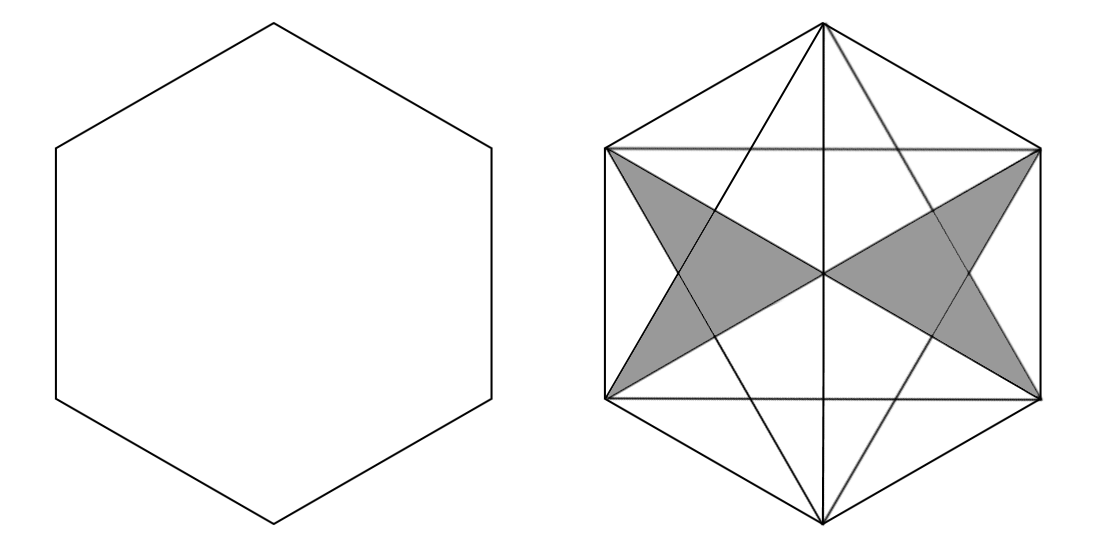# Horrible Hexagons #3The diagram above shows a regular hexagon ${ H }_{3 }$ with area $H$ which has six right triangles inscribed in it. Let the area of the shaded region be $S$, then what is the ratio $H:S?$

×